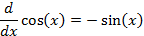Derivatives of Trigonometric Functions

DERIVATIVE OF THE SINE FUNCTION

We start with the sine function and calculate a change in “y” caused by a change in “x” as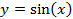And recalling the equation for the sine function for the sum of two angles, we can write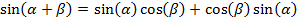And we can multiply the following expression by the same term on the numerator and denominatorAnd remembering the Pythagorean Theorem, we can simplify further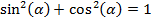Then inserting this term into that for the change in “y” we have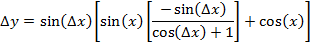Then the derivative operation for the sine function isAnd from simple geometric considerations, the limit of the sine function divided by the change in “x” isSo that finally we haveDERIVATIVE OF THE COSINE FUNCTION

In a similar manner, we can calculate the change in “y” for a change in “x” for the cosine function as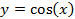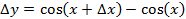And the equation for the cosine function for the sum of two angles we have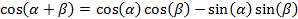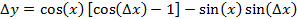And we can substitute the expression above to get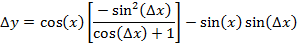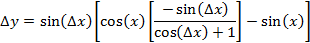The derivative operation for the cosine function is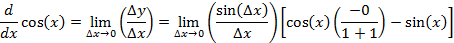which, as above, is finally given as